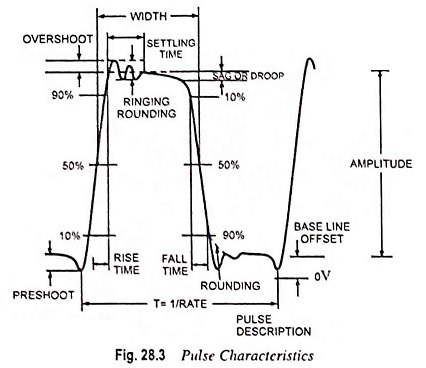## Pulse Characteristics and Terminology:

For describing the output of pulse generators and the applications in which they are used, the introduction of terminology associated with the pulses is very important. The first group of such terms denotes the pulse characteristics of ideal rectangular pulses whereas the second group provides measures of the deviation from the shape and periodicity of ideal pulses.

1. Period is defined as the time duration in seconds between the start of one pulse and the start of the next one.
2. Frequency (or pulse repetition frequency) is the reciprocal of the period.
3. Amplitude is defined as the peak value of the voltage and polarity of the pulse.
4. Pulse width is defined in terms of time duration of the pulse in seconds.
5. Duty Cycle is defined as the ratio of the pulse width to the period or pulse repetition time. It is expressed in per cent of the period. The duty cycle for square waves is of 50 per cent.

However, real pulses and pulse trains only approximate the pulse characteristics of their ideal counterparts. So some additional terms are used for describing the non-ideal aspects of real pulses. These terms along with their definitions are given below and illustrated in Fig. 28.3.The baseline is referred to the dc level and is the line at which the pulse starts and ends. The shift of this line from zero volt, or the expected value is called the baseline offset.

The amplitude of the pulse is measured from the baseline to the steady state value of the pulse.

1. Rise Time: The time the pulse takes to increase from 10 per cent to 90 per cent of its normal amplitude is called the rise time.
2. Fall Time: The time the pulse takes to decrease from 90 per cent to 10 per cent of its normal amplitude is called the fall time.
3. Overshoot is the extent, in terms of per cent of pulse normal amplitude, to which the pulse surpasses its correct value during the initial rise.
4. Ringing is the positive and negative peak distortion, excluding overshoot. It is expressed in per cent of pulse amplitude.
5. Sag or Droop is the fall, in terms of per cent of pulse amplitude, in the pulse amplitude taking place during the pulse width.
6. Pulse Jitter is a measure of short term instability of one event with respect to another, such as instability in the starting time, the pulse width or the pulse amplitude. It is usually expressed as a percentage of the main parameter.
Scroll to Top